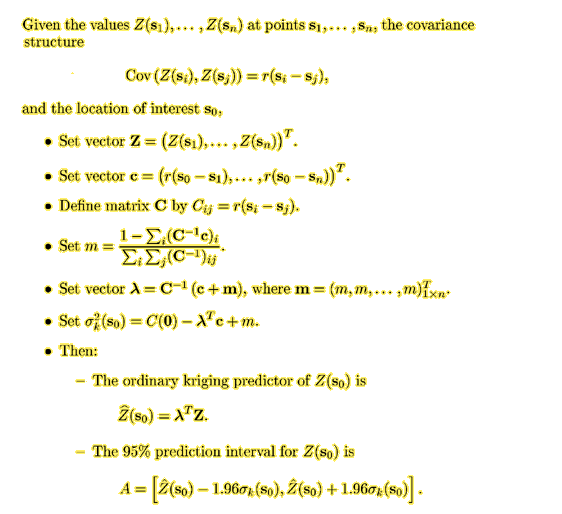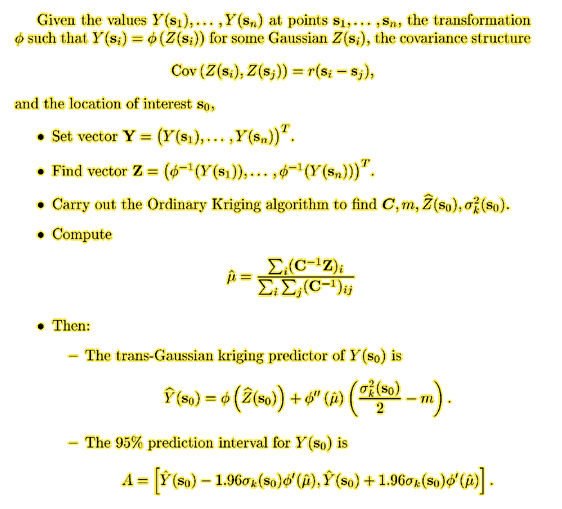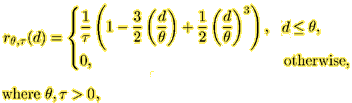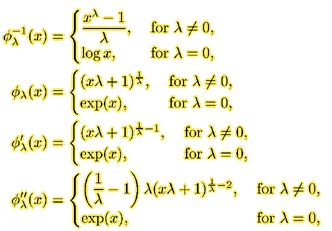# Kriging In Splus

Boris & Sandra Kozintsev, Benjamin Kedem, Department of Mathematics, University of Maryland, 1999.

This web page contains algorithms for Ordinary and Trans-Gaussian Kriging (see Cressie (1993), Statistics for Spatial Data, Wiley: New York, p.123, 137), and their Splus implementation.

## Ordinary Kriging## Trans-Gaussian Kriging## An Splus Implementation

The ordinary kriging is a particular case of the trans-Gaussian kriging when the transformation function is a transaltion, phi(x)=x+c. Therefore we implement the second algorithm only.

The covariance function r is defined as function(x, y, theta1, theta2, tau). For example, for the Spherical covariance functionthe corresponding Splus code is
 sphercov <- function (x, y, theta1, theta2, tau) {         d <- sqrt (x^2 + y^2)         ifelse (d < theta1,              (1- 1.5*(d/theta1)+0.5*(d/theta1)^3) / tau,              0)       }
Note that the covariance function must be able to accept vectors for x and y.

The transformation function, its inverse, and its two derivatives are defined as function(x, lambda). For example, for the Box-Cox model,the corresponding Splus code is
 phiInv <- function (x, lambda)      if (lambda==0) log(x) else (x^lambda-1)/lambda      phi <- function(x, lambda)      if (lambda==0) exp(x) else (x*lambda+1)^(1/lambda)      phiPrime <- function (x, lambda)      if (lambda==0) exp(x) else (x*lambda+1)^(1/lambda-1)      phiDouble <- function (x, lambda)      if (lambda==0) exp(x) else         lambda * (1/lambda - 1) * (lambda * x + 1)^(1/lambda-2)
Note that selecting lambda=1 in this model means using the ordinary kriging.

To perform kriging, the observed data must stored in the form of an n x 3 matrix, where each row represents an (x, y, z) point.

Then, the following function performs the trans-Gaussian kriging. The x- and y-coordinates of the locations at which the prediction is requested are passed to the function in the parameters sx and sy respectively.

 kriging <- function(sx, sy, data, covfun, theta1, theta2, tau, lambda=1) {      if (length(dim(data)) != 2 | dim(data) != 3)                    stop("\nData must be an Nx3 matrix!")      lensx <- length (sx)      if (length(sy) != lensx) stop("\nsx and sy must be same length!")      n <- dim(data)      x <- c(data[,rep(1:2, n)])      y <- c(t(data[,c(rep(1,n), rep(2,n))]))      dif <- matrix(t(matrix(x - y, ncol=2*n)), ncol=2, byrow=T)      C <- matrix (covfun(dif[,1], dif[,2], theta1, theta2, tau), nrow=n)      Cinv <- solve(C)      sumCinv <- sum(Cinv)      Z <- c(phiInv(data[,3], lambda))      mu <- sum (Cinv %*% Z)/sumCinv      prediction <- numeric(lensx)      leftend <- numeric(lensx)      rightend <- numeric(lensx)      for (i in 1:lensx) {        vectorc <- covfun(data[,1]-sx[i], data[,2]-sy[i], theta1, theta2, tau)        m <- (1 - sum (Cinv %*% vectorc)) / sumCinv        vectorlambda <- c((vectorc + m) %*% Cinv)        sigma2 <- covfun(0, 0, theta1, theta2, tau) -                  sum(vectorlambda*vectorc) + m        prediction[i] <- phi(sum(vectorlambda*Z), lambda) +                          phiDouble(mu, lambda) * (sigma2/2 - m)        leftend[i] <- prediction[i] - 1.96*phiPrime(mu, lambda)*sqrt (sigma2)        rightend[i] <- prediction[i] + 1.96*phiPrime(mu, lambda)*sqrt (sigma2)      }      list (prediction=prediction, leftend=leftend, rightend=rightend)   }
The function returns a list of point predictors and left and right ends of the 95% prediction intervals.

Suppose that you have a text file with x, y, z data. For example, run our on-line field generation script (another window will open), and "Save As" the file under the grey-level picture on the left into the directory from which you started Splus, under a name field.txt.

To perform the ordinary kriging prediction at location (4, 6) assuming a Spherical(4, 1, 1) covariance model, type
 data <- matrix(scan(file="field.txt", skip=1), byrow=T, ncol=3)     kriging(4, 6, data, sphercov, 4, 1, 1)

The trans-Gaussian kriging under the Box-Cox model requires positive data. We can shift the observations up by 10, and perform the trans-Gaussian kriging with lambda=0.5:
 data[,3] <- data[,3] + 10     kriging(4, 6, data, sphercov, 4, 1, 1, 0.5)

## Prediction on the Grid

The kriging function above can predict several points at a time by accepting vectors for sx and sy. Therefore, to predict on the grid, one only has to create the vectors with x- and y- coordinates of the points of the grid.

Start by enumerating the projections of the grid on the X and Y axis, for example
 x <- seq (from=0, to=5, by=0.5)     y <- seq (from=0, to=3, by=0.5)

Now create a grid as the cartesian product of x and y by typing
 grid <- cbind(c(matrix (rep (y, length(x)), ncol=length(y), byrow=T)),                 rep (x, length(y)))

To predict on the grid and save the point predictors to a variable called surface, type
 surface <- kriging (grid[,1], grid[,2], data,                                 sphercov, 4, 1, 1)\$prediction

To view the prediction surface, type
 persp (x, y, matrix(surface, ncol=length(y)))

To compare the prediction to the original (observed) surface, type
 persp (matrix(data[,3], ncol=4))

## Saving Prediction Results to a Text File

To save the results of the kriging in the example above, type
 write (t(cbind(grid, surface)), file = "pred3.txt", ncolumns=3)
This creates a file pred3.txt in the directory from which you started Splus with the data in the (x, y, z) form.

If you want to output Z values only, type
 write (t(surface), file = "predZ.txt")